# Grid to Contours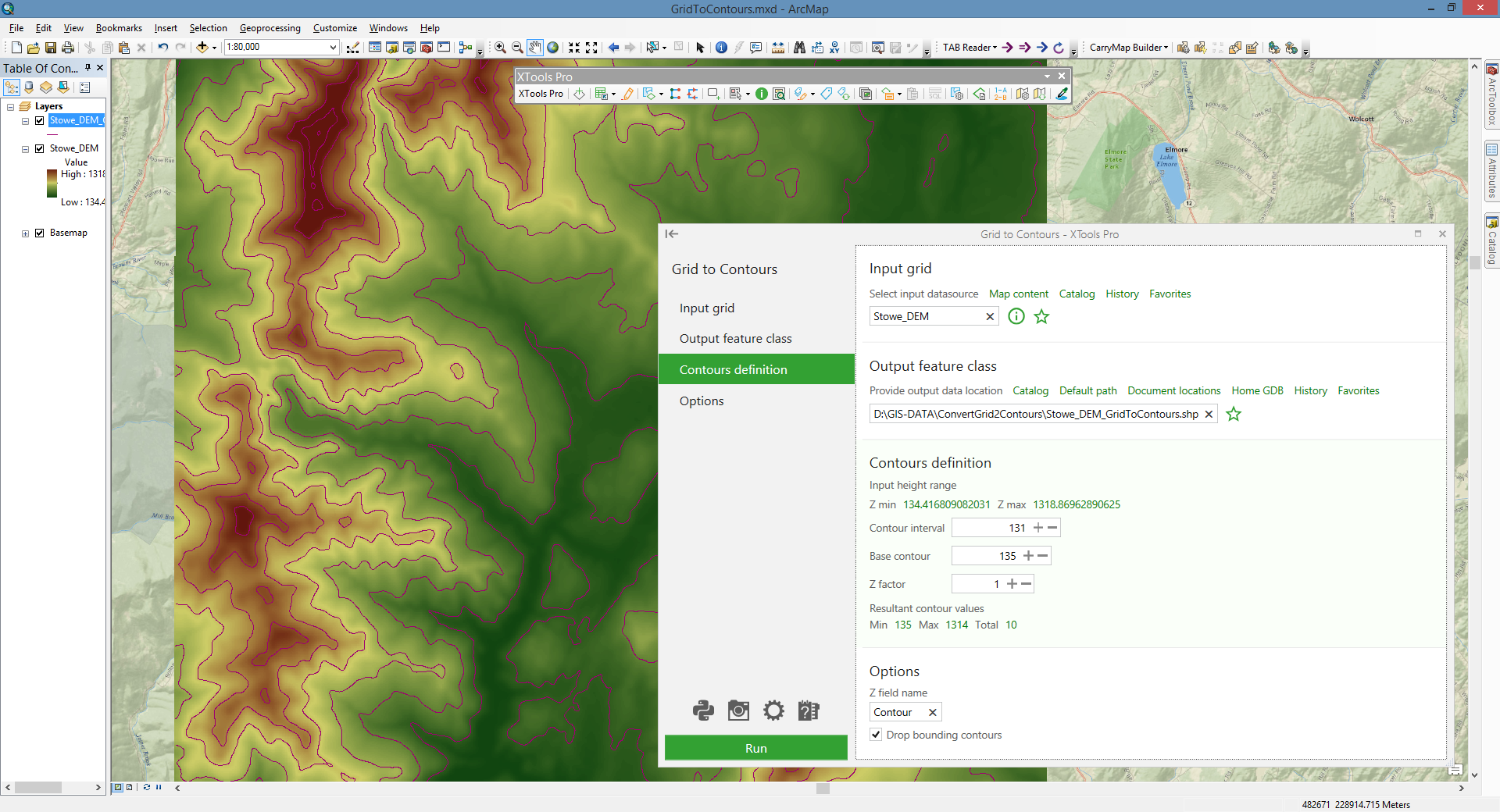Converts raster data models (grids) to contour polyline features (relief isolines, for example) with specified parameters.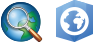Available in XTools Pro for ArcMap and for ArcGIS Pro

The XTools Pro "Grid to Contours" tool is provided to convert raster data models (grids) to contour (relief isolines, for example).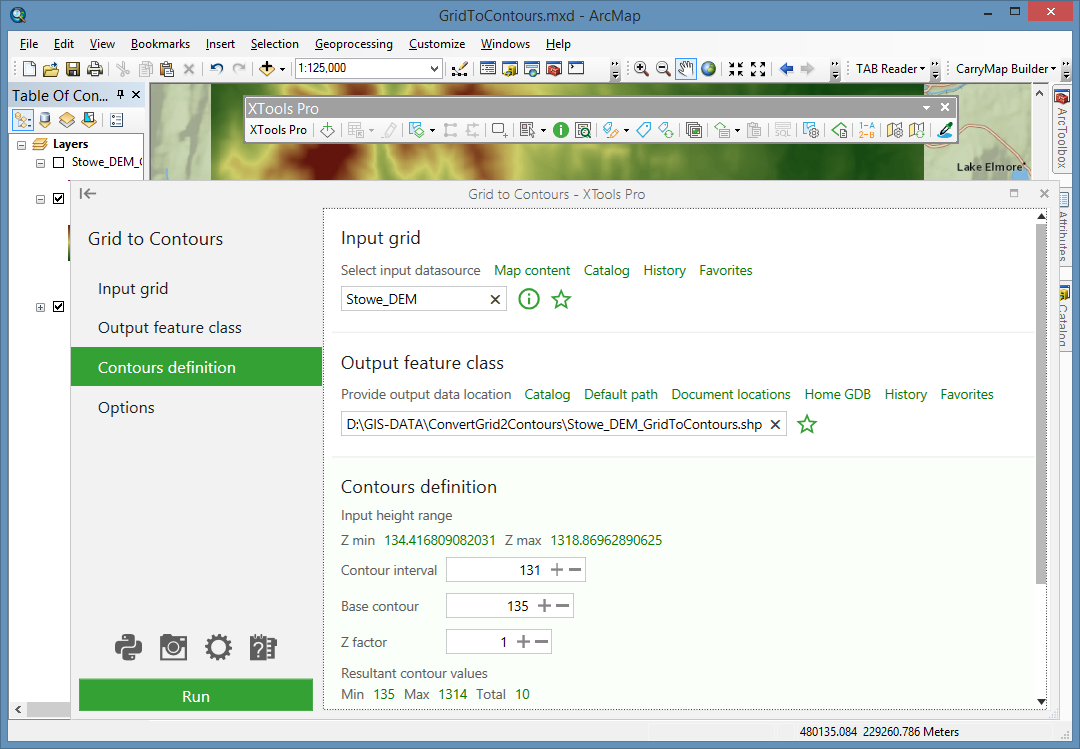Converting raster representation (grid) is performed by defining isoline parameters. It should be noted that one grid may have one or several bands. Only one band of a grid can be converted to contours in a single operation of the tool.Contour is a line that connects points of equal elevation value on a terrain surface. Grids use raster model of geographic data storage. These two representations of geographic data are inter-convertible.

Parameters determined for output contours:

• Contour interval — interval between two neighboring polylines. Default value of the contour interval is calculated taking into consideration that the number of contours between Z min and Z max values must be equal to the Total contour value parameter, which is pre-defined and can be changed in the “Customize XTools Pro Components” dialog. Changing the contour interval value leads to the total contour values number recalculation.
• Base contour — determines the bottom bound of the contour representation. By default its value is equal to the value of the Z min parameter. Changing the base contour leads to recalculation of the total contour values number.
• Z factor — a Z scaling multiplier, which, being not equal to 1, changes Z ranging of a contour representation. The base contour, being multiplied the Z Factor, gives the Minimum contour (an output parameter).  The Z max, being multiplied the Z Factor, gives the Maximum contour (an output parameter).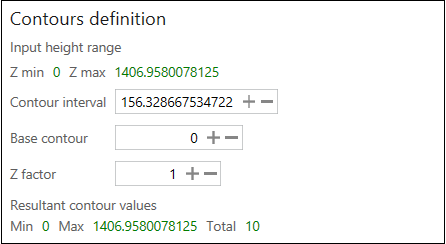For your convenience, information about the input height range (Z min and Z max) is provided.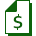Paid tool. Try this and all other XTools Pro features free for 14 days or buy XTools Pro license now.